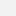Sie sind hier: Klaus Mecke: Space-Time-Matter: …

# Klaus Mecke: Space-Time-Matter: Physics based on Finite Projective Geometry

Klaus Mecke, FAU Erlangen-Nuernberg: Space-Time-Matter: Physics based on Finite Projective Geometry
Wann 19.06.2023 von 17:15 bis 18:00 Großer HörsaalvCaliCal

Modern physics is based on the assumption that natural phenomena are consequences of force fields and elementary particles moving in a continuous spacetime, whose dynamics can mathematically be described by differential equations. An alternative to this substance ontology is the assumption that phenomena are processes of elementary events, which are causally related so that space, time and matter properties emerge from fundamental process relations. This process ontology - proposed by Alfred North Whitehead - can mathematically be formulated as a finite projective geometry of event points where the dynamics is simply
given by local quadratic forms, i.e. by a metric field.

The task in physics remains to derive from this geometric structure the dynamical laws, which are known to be empirically adequate. To this end finite projective analogs of classical mechanics (time dependence), electrodynamics (spatial gauge fields) and quantum mechanics (random particle events) are formulated and their equivalence to the standard (analytical) theories is shown in the continuum limit. The origin of such
important concepts as Legendre transform, gauge symmetry and commutator relations can be elucidated by basic features of finite projective geometry.

Finally, the possibility is outlined of a unified theory of general relativity and quantum field theory of elementary particles, where finite projective geometry is the basic structure instead of a differentiable Riemannian manifold.

Siehe auch hier.